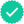Question

Bob Nale is the owner of Nale’s Quick Fill. Bob would like to estimate the mean number of gallons of gasoline sold to his customers. Assume the number of gallons sold follows the normal distribution with a population standard deviation of 1.90 gallons. From his records, he selects a random sample of 45 sales and finds the mean number of gallons sold is 5.70.

 a. What is the point estimate of the population mean? (Round your answer to 2 decimal places.)

 Point estimate

 b. Determine a 90% confidence interval for the population mean. (Use z Distribution Table.) (Round your answers to 2 decimal places.)

 Confidence interval and
Expert SolutionStudents who’ve seen this question also like:Your question is solved by a Subject Matter ExpertWant to see this answer and more?
Experts are waiting 24/7 to provide step-by-step solutions in as fast as 30 minutes!*
*Response times may vary by subject and question complexity. Median response time is 34 minutes for paid subscribers and may be longer for promotional offers.8+ million solutions